American Journal of Civil Engineering
Volume 4, Issue 4, July 2016, Pages: 149-158

Research on Pore Water Pressure of Saturated Muck Soil Under Traffic Loading of Low Frequency

Luo Qiyang, Liu Yongjian, Zhou Yaoyao, Wu Jiansheng, Xie Zhikun

School of Civil and Transportation Engineering, Guangdong University of Technology, Guangzhou, China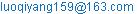(Luo Qiyang)

Luo Qiyang, Liu Yongjian, Zhou Yaoyao, Wu Jiansheng, Xie Zhikun. Research on Pore Water Pressure of Saturated Muck Soil Under Traffic Loading of Low Frequency. American Journal of Civil Engineering. Vol. 4, No. 4, 2016, pp. 149-158. doi: 10.11648/j.ajce.20160404.14

Received: April 10, 2016; Accepted: May 30, 2016; Published: June 2, 2016

Abstract: In order to study the pore water pressure of saturated muck soil under traffic loading of low frequency in Pearl River Delta, the influence of loading frequency, cyclic stress and consolidation on pore water pressure are analyzed. The results show that the final pore water pressure is increased as the frequency decreases, but the increasing rate of pore water pressure is periodical. Moreover, isotropic consolidation can improve the effective stress of soil and slow down the development of pore water pressure, but it is not the key factor. The key factor of influencing the pore water pressure is the cyclic stress, the following is loading frequency, and the consolidation is the last one. In the end, considering cyclic stress, loading frequency and consolidation, a model of pore water pressure based on a math model is built up.

Keywords: Muck Soil, Low Frequency, Traffic Load, Pore Water Pressure, Pore Pressure Model

1. Introduction

2. The Dynamic Triaxial Test of Saturated Muck Soil Under Traffic Loading of Low Frequency

2.1. Experiment Apparatus and Soil Sample

A dynamic test of saturated muck soil is carried out by GCTS SPAX-2000 (modified) static and dynamic true triaxial testing system which is developed and manufactured by United States. The system consists of seven sub-systems: (1) SPAX-100 true triaxial pressure chamber; (2) PCP-2000-H pressure control panel; (3) PVC-25-HC confining pressure / volume computer servo controller; (4) PVC-200-HP back pressure / pore-pressure volume controller; (5) SCON-2000 digital servo controller and acquisition system; (6) HPS-10-5 variant constant pressure pump; (7) WIN -CATS-ADV control and post-processing software. The entire testing process is controlled by integrated computer software named GDSLAB that can collect and deal with the data to emulate a partial stress and cyclic stress traffic load.

The soft soil sample was taken from Shenzhen Baoan Airport, which is in offshore sea level 12m~15m. It contains mica, quartz and shell debris. The moisture content and compressibility are high and the permeability is poor. Basic physical and mechanical properties of samples are shown in Table 1.

Table 1. Physical and mechanical properties of soft clay.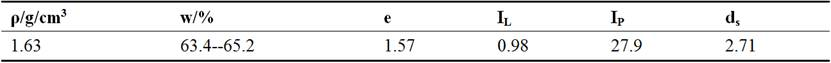2.2. Testing Program

Isotropic and anisotropic consolidation are took in this experiment. The frequency are 0.1Hz, 0.25Hz, 0.5Hz and 1 Hz. The cyclic stress are 20 kPa, 50 kPa and 65kPa. The test program is shown as Table 2. εd =15% is the failure standard. If the soil sample did not fail, the sample would be shear by 0.01%/min until broken. In order to simulate actual operation, firstly, the drain valve is going to be closed before dynamic loading. And then the initial static deviator stress is loaded, which is half of dynamic stress.

Table 2. Un-drained cyclic triaxial test parameters.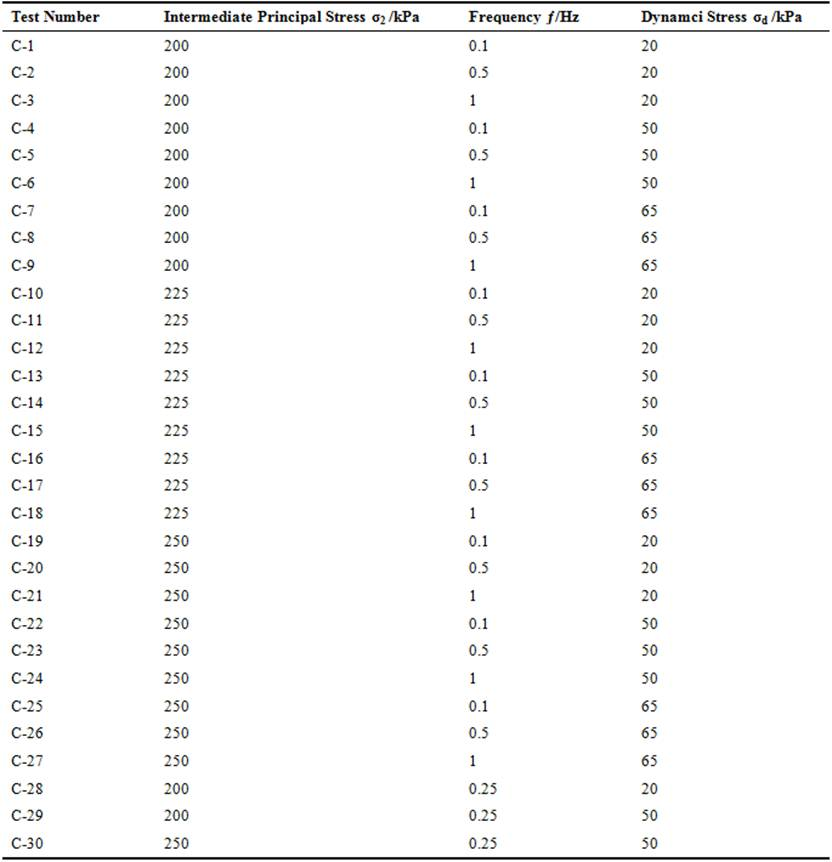3. Test Result and Analysis

3.1. The Law of Super Pore Water Pressure Under Different Frequencies

The curves of the relationship between super pore water pressure u and cyclic number N under different frequency of the same dynamic stress and isotropic consolidation σ1′=σ2=σ3=200kPa are shown in Figure 1, 2, 3. The curves of the relationship between super pore water pressure u and cyclic number N under different frequency of the same dynamic stress and anisotropic consolidation σ1′=σ2=225kPa, σ3′=200kPa are shown in Figure 4, 5, 6. The curves of the relationship between super pore water pressure u and cyclic number N under different frequency of the same dynamic stress and anisotropic consolidation σ1′=σ2=250kPa, σ3′=200kPa are shown in Figure 7, 8, 9.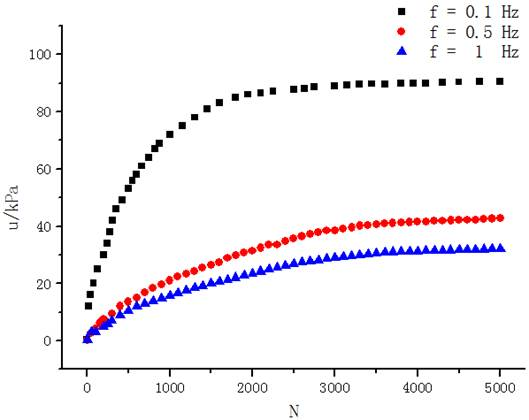Effective stress σ1′=σ2′=σ3′=200kPa, dynamic stress σd=20kPa

Figure 1. Super Pore Water Pressure u against Cyclic Number N.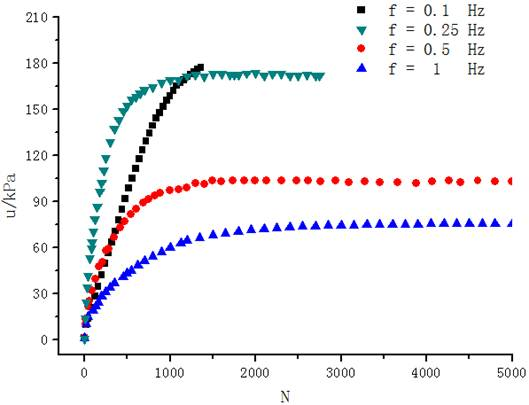Effective stress σ1′=σ2′=σ3′=200kPa, dynamic stress σd=50kPa

Figure 2. Super Pore Water Pressure u against Cyclic Number N.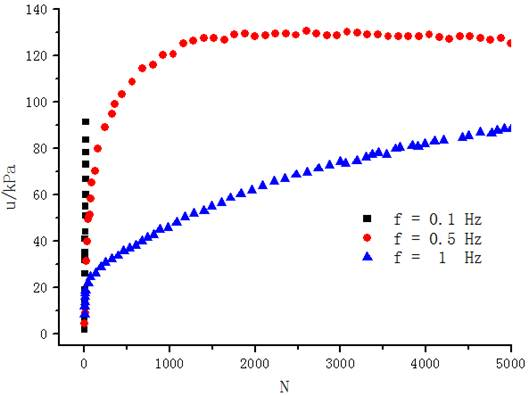Effective stress σ1′=σ2′=σ3′=200kPa, dynamic stress σd=65kPa

Figure 3. Super Pore Water Pressure u against Cyclic Number N.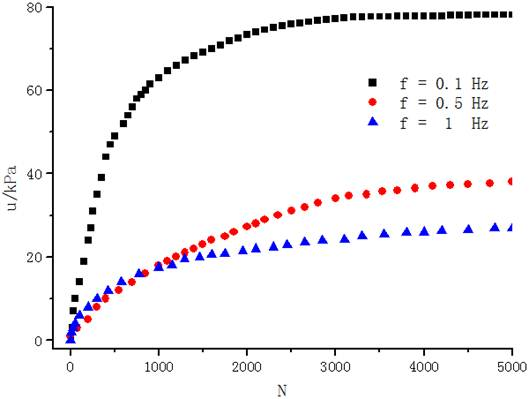Effective stress σ1′=σ2′=225kPa, σ3′=200kpa, dynamic stress σd=20kPa

Figure 4. Super Pore Water Pressure u against Cyclic Number N.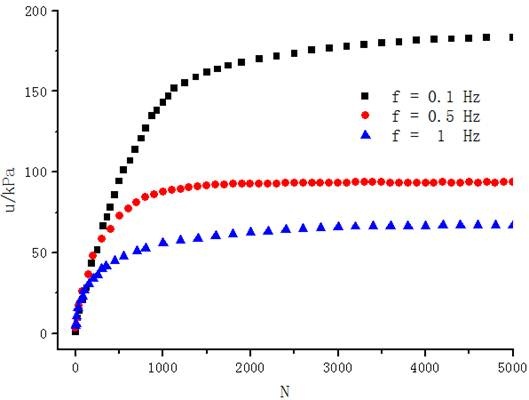Effective stress σ1′=σ2′=225kPa, σ3′=200kpa, dynamic stress σd=50kPa

Figure 5. Super Pore Water Pressure u against Cyclic Number N.Effective stress σ1′=σ2′=225kPa, σ3′=200kpa, dynamic stress σd=65kPa

Figure 6. Super Pore Water Pressure u against Cyclic Number N.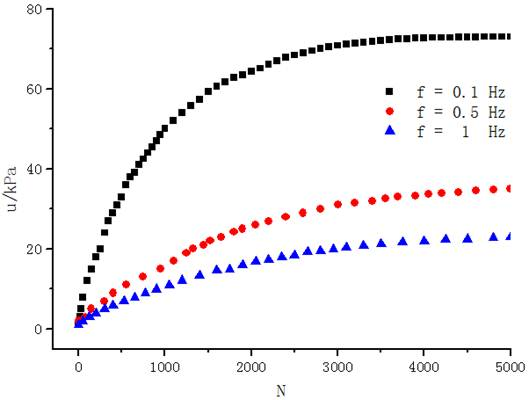Effective stress σ1′=σ2′=250kPa, σ3′=200kpa, dynamic stress σd=20kPa

Figure 7. Super Pore Water Pressure u against Cyclic Number N.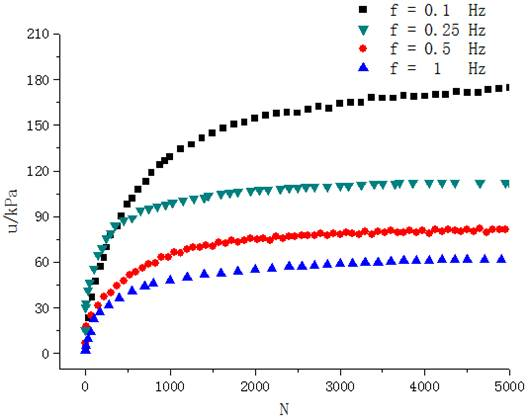Effective stress σ1,2′=250kPa, σ3′=200kpa, dynamic stress σd =50kPa

Figure 8. Super Pore Water Pressure u against Cyclic Number N.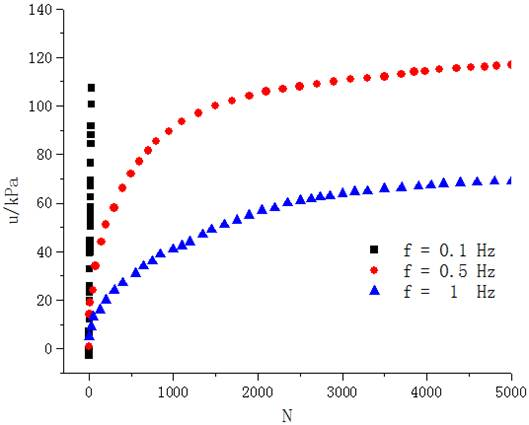Effective stress σ1′=σ2′=250kPa, σ3′=200kpa, dynamic stress σd =65kPa

Figure 9. Super Pore Water Pressure u against Cyclic Number N.

Since this soil samples are taken from the offshore plane following 13m~16m which lead to that the moisture content is higher than 63% generally, some of them are as high as 68%, which result in that the dynamic property is poorer than common clay. The effective stress of the sample was still lower than the similar saturated clay under the same condition even after 96 hours solidifying. Therefore the majority of energy is undertaken by pore water of the soil body during the initial period loading. No matter the growth or the final value of pore-water pressure are, this soil sample is faster and higher than the common saturated clay, which is shown in Figure 1~9.

Figure 1 shows the change of the super pore water pressure of the soil sample which is consolidated after 200kPa and then loaded by different frequencies and dynamic stress of 20kPa. Taking the 0.1Hz for example, in the initial loading period (the cyclic numbers are about 2000), the energy is mainly undertaken by the soil body and the pore water, therefore the pore water pressure rapidly grows and reaches as high as 77% of the limiting super pore water pressure. Along with the soil body compacting and the particles between the pore reducing gradually, the main pore water is working and the pore water pressure’s growing rate slows down obviously. And then the pore water pressure gradually becomes stable and stay in a stage which it almost does not grow. That is called the limiting value of the pore-water pressure, which is the 45% of the effective confining pressure. Generally, the growth of the super pore water pressure can be summarized as three stages---rapid growth, slow growth and gradual staying, which is similar to the study result of the saturated soft soil around tunnel under cyclic loading by Tang Yiqun .

The comparison of super pore pressure water of 50kPa under 0.1Hz, 0.25Hz, 0.5Hz and 1Hz is shown in Figure 2. Comparing to the 20kPa, the final pore water pressure of 50kPa is higher than the former. That is because the higher the cyclic stress is, the higher energy impacting to the soil, which leads to that the higher energy can be absorbed by pore water under the same condition. Comparing to the 0.1Hz and 0.25Hz, although the final pore water pressure of 0.25Hz is closed to 0.1Hz, which presents that the influence of frequency on the final pore water pressure is weaken when the frequency is low enough, the increasing rate of 0.25Hz is still larger than 0.1Hz, which is closed to the result of Yasuhara , a study showing that the higher the frequency is, the higher the increasing rate of pore water pressure become. However, when the frequency is larger than 0.25Hz, the growth of the pore water is closed to the viewpoint of most scholars, like Wang Jun and Zhou Nianqing [11,12], which suggested that the lower the frequency is, the higher the increasing rate of pore water pressure and the higher final pore water pressure become. This results from that lower frequency means the more time of the load working on soil body. The more energy on the soil are, the higher the final pore water pressure become since the pore water pressure become accumulative gradually and can not dissipated quickly under the same loading frequency. Therefore under the low frequency cyclic loading, the increasing rate of the pore water pressure of saturated muck soil appears periodical. During the frequency of 0.1Hz~0.25Hz, the relationship between frequency and the pore water pressure is related, namely that the higher the loading frequency is, the higher the increasing rate of pore water pressure become. However, between the frequency of 0.25Hz~1Hz, the relationship between frequency and the pore water pressure is negative, namely the higher the frequency is, the lower the increasing rate of the pore water pressure become.

Figure 3, 6, 9 shows the change of the pore water pressure of cyclic stress 65kPa under different consolidation stresses. The increasing type of the pore water pressure is the rapidly rising under 0.1Hz, which presents that the pore water pressure keep an high increasing rate until the soil to be broken from the beginning of the load to the end. According to the information above, the rapidly rising type of pore water pressure mostly happens in low frequency and high cyclic stress. The type of stable mostly happens in high frequency and low cyclic stress. And the type of gradually growing is the transitional stage between type of rapidly rising and stable.

Figure 8 shows that how the pore water pressure grow during different frequency, like 0.1Hz, 0.25Hz, 0.5Hz and 1Hz, which is different to the result above. The final pore water pressure do not increase largely as the frequency, but grow slowly after 0.5Hz. That suggests that there is an threshold of frequency between 0.1Hz~1Hz, leading to the type changing of how the pore water pressure increase. The increasing rate of pore water pressure slow down when the experimental frequency is higher than the threshold.

3.2. The Law of Super Pore Water Pressure Under Different Dynamic Stress

The curves of the relationship between super pore water pressure u and cyclic number N under different dynamic stress of the same frequency and isotropic consolidation σ1′=σ2=σ3=200kPa are shown in Figure 10, 11, 12. The curves of the relationship between super pore water pressure u and cyclic number N under different dynamic stress of the same frequency and anisotropic consolidation σ1′=σ2′=225kPa, σ3′=200kPa is shown in Figure 13, 14, 15. The curves of the relationship between super pore water pressure u and cyclic number N under different dynamic stress of the same frequency and anisotropic consolidation σ1′=σ2=250kPa, σ3′=200kPa are shown in Figure 16, 17, 18.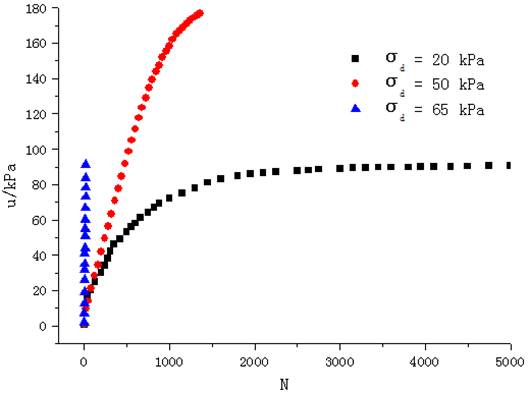Figure 10. Super Pore Water Pressure u against Cyclic Number N.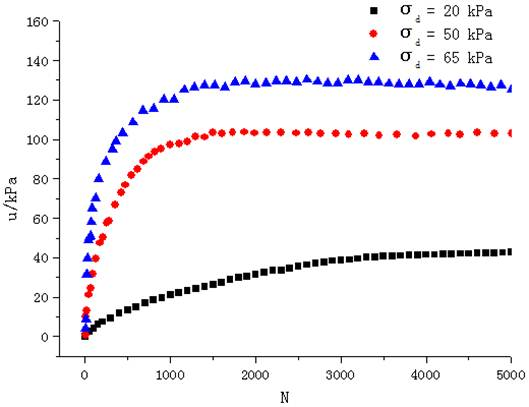Figure 11. Super Pore Water Pressure u against Cyclic Number N.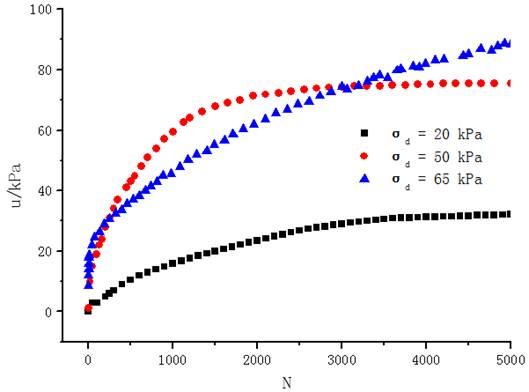Figure 12. Super Pore Water Pressure u against Cyclic Number N.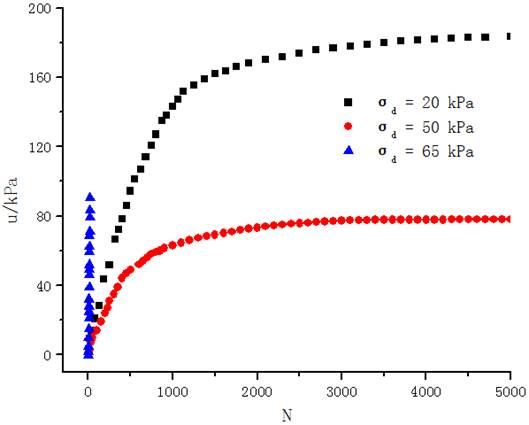Figure 13. Super Pore Water Pressure u against Cyclic Number N.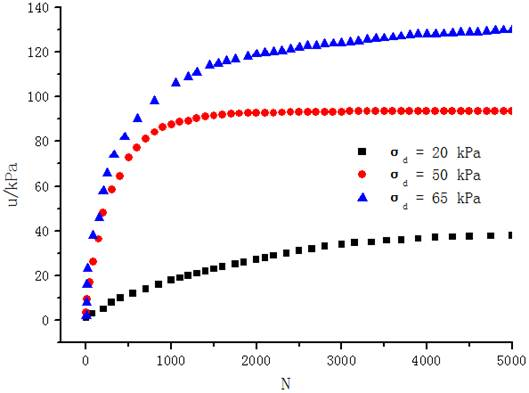Figure 14. Super Pore Water Pressure u against Cyclic Number N.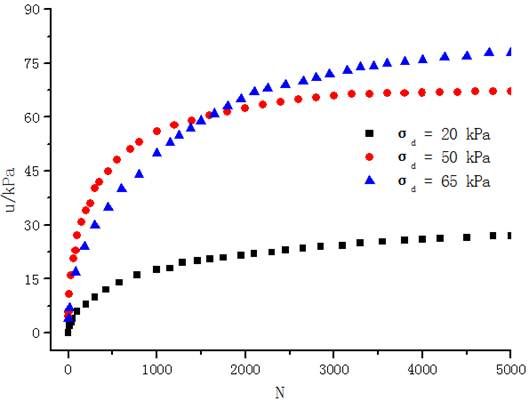Figure 15. Super Pore Water Pressure u against Cyclic Number N.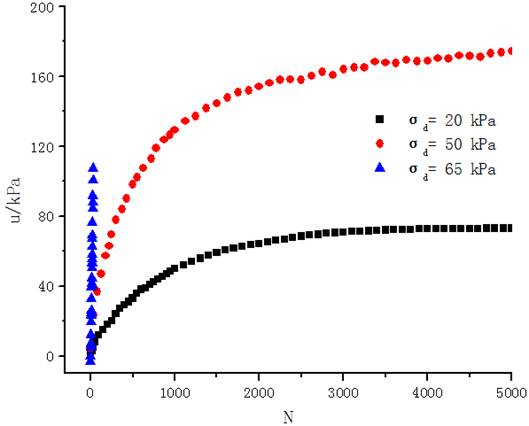Figure 16. Super Pore Water Pressure u against Cyclic Number N.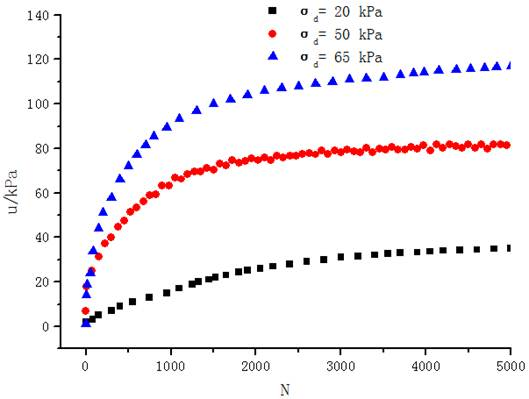Figure 17. Super Pore Water Pressure u against Cyclic Number N.Figure 18. Super Pore Water Pressure u against Cyclic Number N.

The curve of the relationship between super pore water pressure u and cyclic number N of different cyclic stress under 0.1Hz and the isotropic consolidation is shown in Figure 10, which presents that the higher cyclic stress is, the higher increasing rate of the pore water pressure become. That is because higher cyclic stress presents more energy working on the soil body. Since the construction of saturated muck soil is poorer than common soil clay, most energy is maintained by the soil pore, which leads to the pore water increasing rapidly. In addition, considering the failure standard is 15%, the final pore water pressure of 65kPa is lower than 50kPa, which means the final pore water pressure is not proportional to the cyclic stress, since there is not enough time for the pore water pressure to increase when the energy loading on the soil body is too great. So the experimental cyclic stress should be less than 50kPa if requiring to study the development of pore water pressure under 0.1Hz according the experimental result.

Even most studies [1~6] show that the higher the cyclic stress are, the quicker the increasing rate of pore water pressure develop, but this is based on a limited cyclic numbers and cyclic stress. Figure 12, 15, 18 show that the instant pore water pressure of 50kPa is higher than 65kPa under the cyclic numbers of 0~2000, which presents that the development of pore water pressure do not follow the rule that the higher the cyclic stress is, the higher the increasing rate of pore water pressure become since the higher cyclic stress are, the quicker for the balance between effective stress and pore water pressure break. However, when the cyclic numbers are larger than 5000, the final pore water pressure still increase as the cyclic stress increase since the more energy of the pore absorb, the higher the final pore water pressure increase.

3.3. The Law of Super Pore Water Pressure Under Different Dynamic Stress

The curves of the relationship between super pore water pressure u and cyclic number N of different consolidation, the same dynamic stress and frequency are shown in Figure 19, 20, 21. The curves of the relationship between super pore water pressure u and cyclic number N of different consolidation, the same dynamic stress and frequency are shown in Figure 22, 23, 24. The curves of the relationship between super pore water pressure u and cyclic number N of different consolidation, the same dynamic stress and frequency are shown in Figure 25, 26, 27.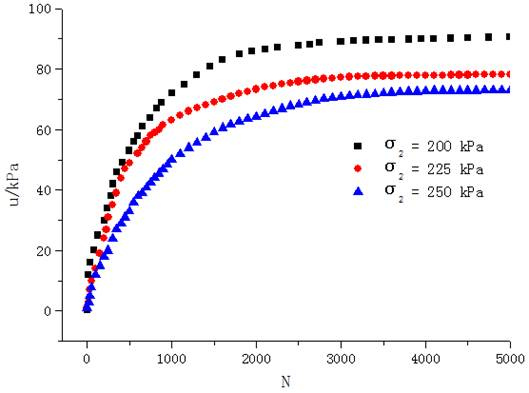Figure 19. Super Pore Water Pressure u against Cyclic Number N.Figure 20. Super Pore Water Pressure u against Cyclic Number N.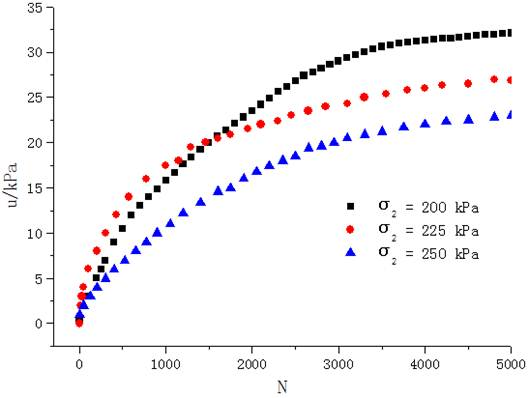Figure 21. Super Pore Water Pressure u against Cyclic Number N.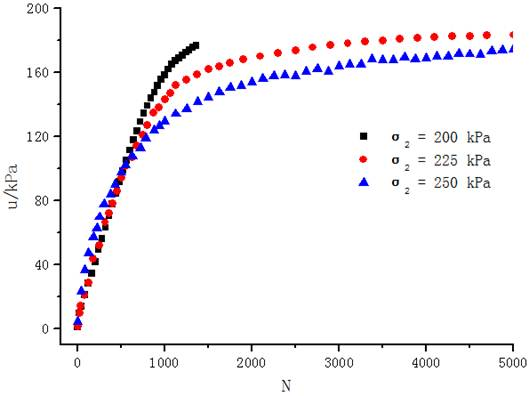Figure 22. Super Pore Water Pressure u against Cyclic Number N.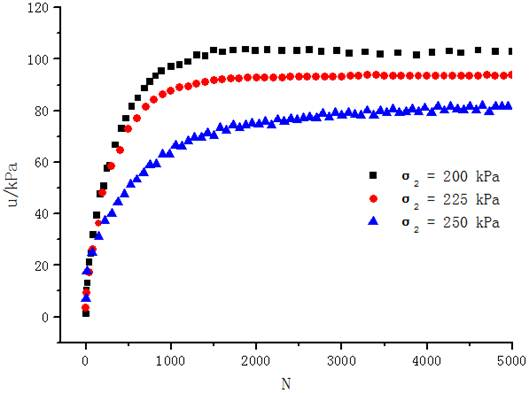Figure 23. Super Pore Water Pressure u against Cyclic Number N.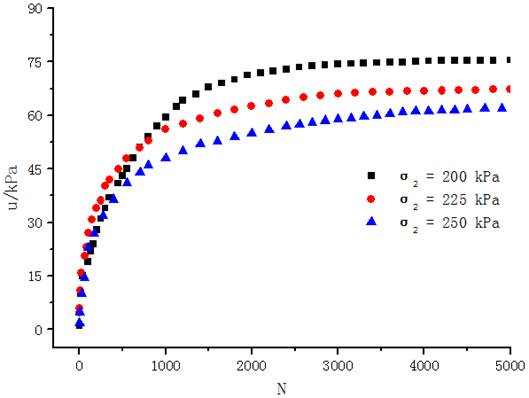Figure 24. Super Pore Water Pressure u against Cyclic Number N.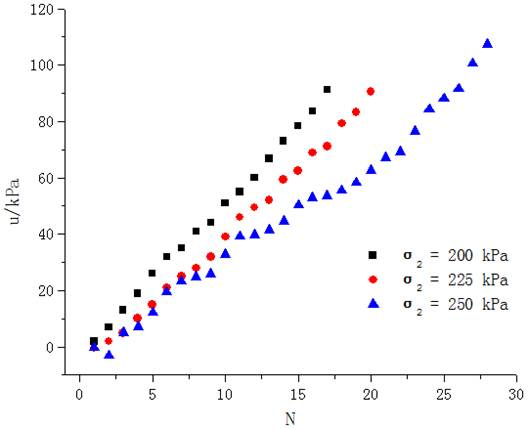Figure 25. Super Pore Water Pressure u against Cyclic Number N.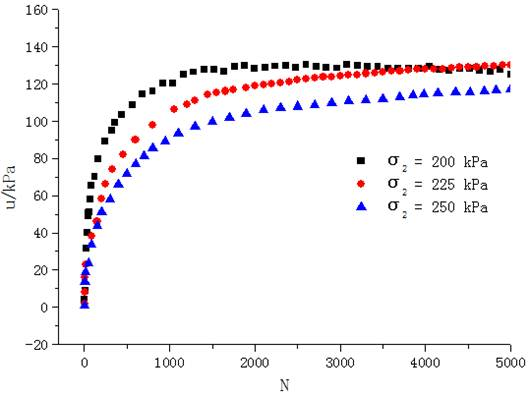Figure 26. Super Pore Water Pressure u against Cyclic Number N.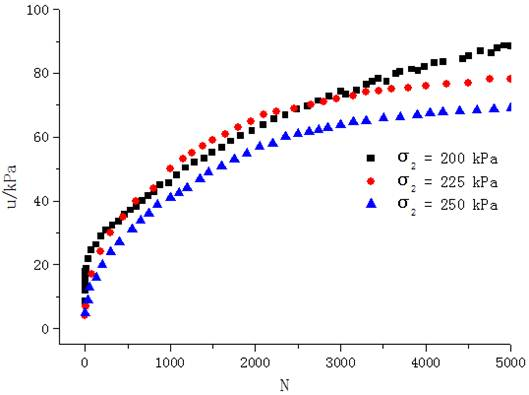Figure 27. Super Pore Water Pressure u against Cyclic Number N.

The curves of the relationship between super pore water pressure u and cyclic number N of different consolidation stress under each loading frequency and 20kPa are shown in Figure 19, 20, 21. Those figures present that anisotropic consolidation lead to the different influence, comparing to loading frequency and cyclic stress, for the construction of soil. For example, since the reconstruction of soil particle leads to the increasing of the effective stress and reconstruction of drainage passage, in the equal dynamic situation, more energy can be undertaken by the construction of soil, which leads to the less energy of the pore water absorbing and the slower of the pore water pressure increasing speed. Moreover, the higher the frequency is, the more obvious the effect of anisotropy present. The comparison of different consolidation stresses under 0.1Hz are shown in Figure 19, which presents that the final pore water pressure of effective stress 250kPa is as high as the 78% of 200kPa, so that of 1Hz 69%. The dates above shows that the higher the frequency under the same consolidation are, the stronger the anisotropy become.

Moreover, Figure 22, 26 shows that the anisotropy also influence the cyclic numbers of soil developing to failure. For example, the development of super pore water pressure of 65kPa under 0.5Hz is shown in Figure 26. In the isotropic consolidation 200kPa, the pore water pressure increase rapidly when the cyclic numbers N are about 3100, which suggests that the construction of soil change. However, as the intermediate principal stress increase, it can impede, even prevent the soil broken.

According to Figure 1~27, under the traffic load of low frequency, the biggest factor affecting pore water pressure is cyclic stress, followed by loading frequency and consolidation stress. This is because although the consolidation stress can change the arrangement of soil particles, promote pore water drainage and improve soil effective stress, many structural changes are fundamentally impossible due to the low structure of the muck soil compared to clay. While at low frequency 0.1Hz ~ 0.5Hz, the time of pore water absorbing the energy from cyclic loading is similar, which weakens the effect of loading frequency. In contrast, from previous studies, cyclic stress is the key factor of pore water pressure rising, which is due to the pore water pressure absorbing energy. Therefore, the cyclic stress is the most critical factor for the growth of the pore water pressure.

4. A New Pore Pressure Model and Its Parameters

Since the development of pore pressure of muck soil under traffic loading is complicated, it is hard to build a comprehensive pore pressure model. In order to practicality, the convenience of variables should be considered firstly when choosing the model type. Yang Ping  suggested that the pore pressure model type of hydraulic is different under low and high frequency. Polynomial is suitable for low frequency while power exponent is suitable for high frequency. Here is the pore pressure model for muck soil in Pear River Delta, which is shown as fellow: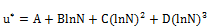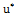is the normalized pore pressure. N is the cyclic number. A, B, C and D are the experimental parameters.

Table 3. Parameters of model.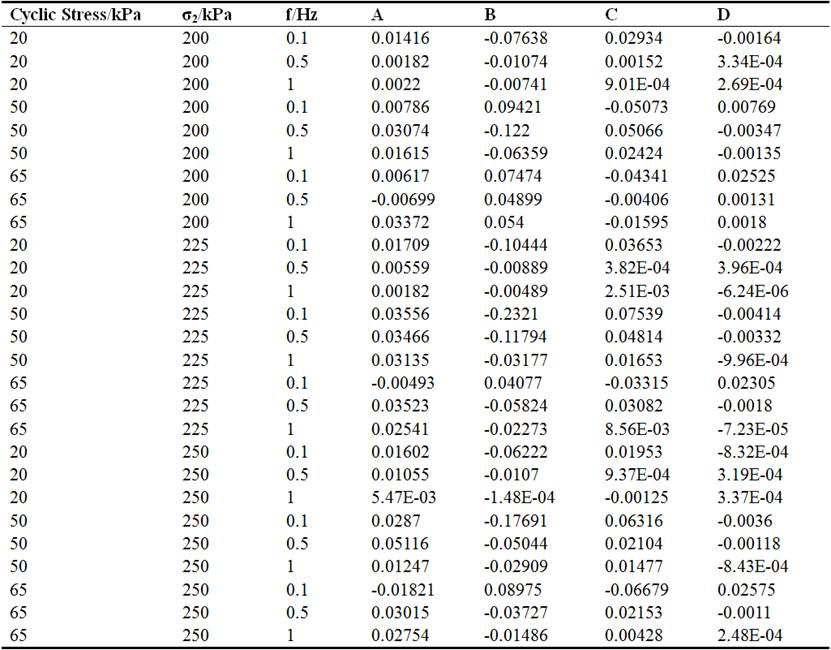5. Conclusion

The author, firstly, summarize the previous studies on pore water pressure under dynamic stress and take a dynamic test of saturated muck soil, which is carried out by GCTS SPAX-2000 (modified) static and dynamic true triaxial testing system. Here are the conclusions:

(1) In the same cyclic stress and consolidation, the final pore water pressure decreases as the increasing frequency.

(2) In the same cyclic stress and consolidation, the influence of loading frequency on the increasing rate of pore water pressure is periodical. In the 0.1Hz~0.25Hz, the increasing rate of pore water pressure is raised by the frequency increasing. However, in the 0.25Hz~1Hz, the increasing rate of pore water pressure decrease as the frequency increase.

(3) In the same loading frequency and consolidation, the higher cyclic stress is, the more quickly the pore water pressure develop, as well as the accumulative pore water pressure.

(4) In the same cyclic stress and loading frequency, isotropic consolidation can increase the effective stress of soil and slow down the development of pore water pressure, but it is not the key factor.

(5) During the loading frequency of 0.1Hz~1Hz, the key factor of influencing the pore water pressure is cyclic stress and the following is the loading frequency, consolidation is the last one.

(6) Considering cyclic stress, loading frequency and consolidation, a model of pore water pressure based on an math model is built up:References

1. Procter, David C., and Jalal H. Khaffaf. Cyclic triaxial tests on remoulded clays [J]. Journal of Geotechnical Engineering, 1984, 110 (10): 1431-1445.
2. Zhou Jian, Gong Xiaonan. Study on strain soften in saturated soft clay under cyclic loading [J]. China Civil Engineering Journal, 2000, 33 (5): 75-78.
3. Ansal, Atilla M., and Ayfer Erken. Undrained behavior of clay under cyclic shear stresses [J]. Journal of Geotechnical Engineering, 1989.
4. Yasuhara Kazuya, Yamanouchi Toyotoshi, Hirao Kazutoshi. Cyclic strength and deformation of normally consolidated clay [J]. Soils and Foundations, 1982, 22 (3): 77-79.
5. Zhang Yu, Tu Yangju, Fei Wenping. etc. Effect of vibration frequency on dynamic properties of saturated cohesive soil [J]. Rock and Soil Mechanics, 2006, 27 (5): 699-704
6. Wei Xinjiang, Zhang Tao, Ding Zhi. etc. Experimental study of pore pressure model of soft clay with different consolidation degrees under subway loading [J]. Rock and Soil Mechanics, 2014, 35 (10): 2761-2768.
7. Wang Jun, Yang Fang, Wu Yanping. etc Experiment study of coupling effect of initial shear stress and loading rate on pore water pressure model of soft clay [J]. Rock and Soil Mechanics, 2011 (S1): 111-117.
8. Wang Yuandong, Tang Yiqun, Liao Shaoming, etc. Experimental of Pore Pressure of Reinforced Soft Clay Around Tunnel Under Subway Vibrational Loading [J] Journal of Jilin University (Earth Science Edition), 2011, 41 (1): 188-194.
9. Ding Zhi, Zhang Tao, Wei Xinjiang, etc. Experimental study on effect of different drainage conditions on dynamic characteristics of soft clay under different degrees of consolidation [J]. Rock and Soil Mechanics, 2015 (5): 893-899.
10. Zhao Chunyan, Zhou Shunhua, Zhuang Li. Cyclic Accumulative Pore Pressure Model of Soft Clay in the Shanghai Region [J] Journal of the China Railway Society. 2012, 34 (1): 77-82.
11. Zhou Nianqing, Tang Yiqun, Wang Jianxiu, etc. Response characteristics of pore pressure in saturated soft clay to the metro vibration loading [J]. Chinese Journal of Geotechnical Engineering, 2006, 28 (12): 2149-2152.
12. Wang Jun, Cai Yuanqiang, Li Xiaobing. Cyclic softening-pore pressure generation model for overconsolidated clay under cyclic loading [J]. Rock and Soil Mechanics, 2008, 29 (12): 3217-3222.
13. Tang Yiqun, Zhang Xi, Zhao Shukai, etc. Model of pore water pressure development in saturated soft clay around subway tunnel under vibration load [J]. China Civil Engineering Journel, 2007, 40 (4): 82-86.
14. Yang Ping, Tang Yiqun, Zhou Nianqing, etc. Study on Pore Water Pressure of Hydraulic Fill under Traffic Loading [J]. Chinese Journal of Underground Space and Engineering, 2008, 4 (2): 253-258.

 Contents 1. 2. 2.1. 2.2. 3. 3.1. 3.2. 3.3. 4. 5.
Article ToolsAbstractPDF(3301K)